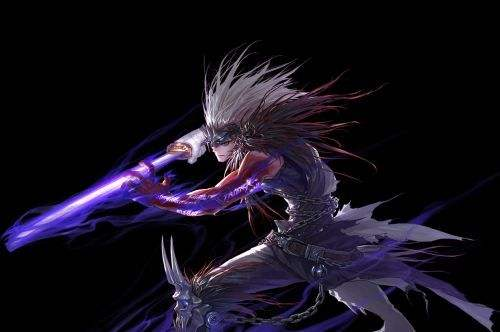说实话本来就没多强，不过策划果然发现45技能加强的有点多了，然后看了看砍的幅度，鱼头二觉都是9%减少，突然发现这次砍的正好是正式服那波爆发加强砍就砍了吧，啥时候能把绿阵白字bug给修复了啊，韩服鬼泣是真的不给力啊，韩服有没有这个bug也不知道百分比提升是实打实的：鱼头提升14.4%(砍后提升4.1%)冥炎剑提升18.4%(砍后削弱1.3%)刀阵终结提升27.5%(砍后提升16%)鞭子几乎0提升原固伤，魔攻/**系数为1的时候提升如下：吉格提升17.3%(砍后提升6.7%)月牙提升6.3%鱼锤提升6%小墓碑提升6%炼狱提升6.3%卡洛提升7.4%(存疑具体数据我没得到，找的别人算的数据估算的)最后正式服砍20%有点伤自身系数算法：站街魔攻/(1+武器精通比如42%就是0.42)/(站街智力/250+1)/站街**=系数X其实除以**之前的那个数你可以手动加40，因为无色小晶体的进图40魔攻并不会站街的时候加成进去最后算出来的系数乘以原固伤的提升就是自身改版后的提升，比如我自己是0.88，吉格提升就是0.88*1.173=1.032，意思是改版后我的吉格至少伤害加3.2%最后提醒下仅为修炼场提升，实际组队刷图部分人最后可以乘以1.15都不为过，毕竟大部分**墓碑暗强实际刷图也没有吃到过，绿阵也没有第一时间柔出来，这次还有个隐藏改版，柔滑没有了内置CD，懂的人应该懂吧，只要你手速够快，一个鱼头能柔4个技能都不是梦是不是觉得这次改版鬼泣伤害并没有得到想象中加强幅度，有很大一部分原因是砍得近100暗强确实有点伤，但实际上这次改版多了两个短CD高输出技能冰阵(15秒)和紫阵(20秒)，某些职业凑8个输出技能都不够的时候，鬼泣伤害高输出技能在12个以上，在SP允许下选其中高的八九个，然后5秒内打完。我觉得还行，最后隐藏一个计算过程(可忽略)：100暗强提升21% 红阵800智力换成24技能攻击力，单刷我大概认为能提升3%，组大奶能提升10%甚至更多鱼头97514/72528=1.3445/1.21=1.111*1.03=1.144冥炎剑71519/51413=1.391/1.21=1.1496*1.03=1.184刀终结55735/37217=1.4976/1.21=1.2377*1.03=1.275鞭子19973/17042=1.172/1.21=0.9686*1.03=1原固伤部分武器精通取42%吉格94497/97362=0.9706*1.42=1.3782/1.21=1.139*1.03=1.173月牙56298/63993=0.8798*1.42=1.2492/1.21=1.0324*1.03=1.063鱼锤36728/41881=0.8769*1.42=1.2453/1.21=1.0292*1.03=1.06炼狱同月牙为1.0324*1.03=1.063小墓碑同鱼锤为1.06卡洛1.2619/1.21=1.043*1.03=1.0742